# MCQ in DC Circuits Part 9 | REE Board Exam

(Last Updated On: November 13, 2020)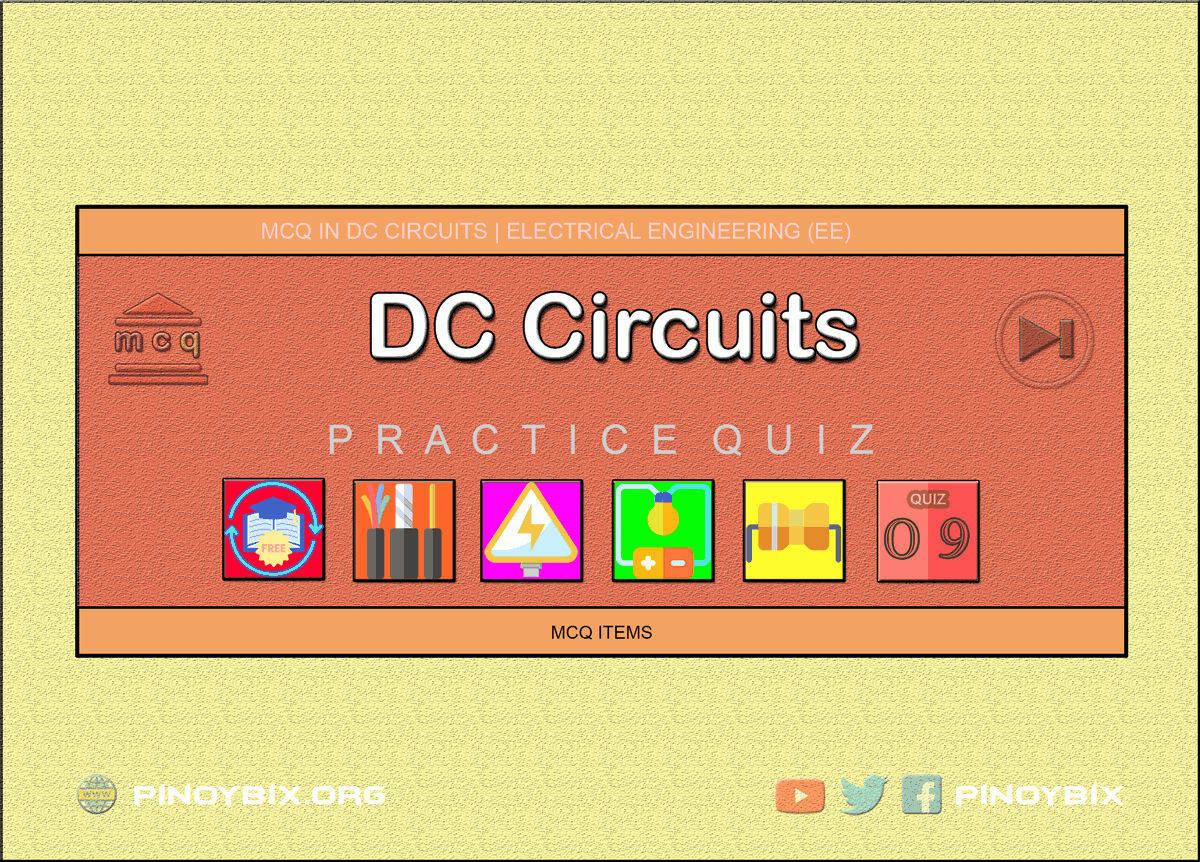This is the Multiple Choice Questions Part 9 of the Series in DC Circuits as one of the Electrical Engineering topic. In Preparation for the REE Board Exam make sure to expose yourself and familiarize in each and every questions compiled here taken from various sources including but not limited to past Board Exam Questions in Electrical Engineering field, Electrical Engineering Books, Journals and other Electrical Engineering References.

#### Continue Practice Exam Test Questions Part 9 of the Series

NETWORK THEOREMS

Choose the letter of the best answer in each questions.

401.  The central terminal of a dry cell is said to be

A.  positive

B.  negative

C.  neutral

D.  charged

Solution:

402.  A  24  V  battery  of  internal  resistance  r  =  4  Ω  is connected  to  a  variable  resistance  R,  the  rate  of heat  dissipation  in  the  resistor  is  maximum  when the  current  drawn  from  the  battery  is  I.  Current drawn from the battery will be I/2 when R is equal to

A.  8 Ω

B.  12 Ω

C.  16 Ω

D.  20 Ω

Solution:

403.  What  is  the  other  term  used  for  rechargeable battery?

A.  primary

C.  storage

Solution:

E.   NETWORK THEOREMS

404.  REE Board Exam April 1997

A  circuit  consisting  of  three  resistors  rated:  10 ohms,  15  ohms  and  20  ohms  are  connected  in delta.  What  would  be  the  resistance  of  the equivalent wye connected load?

A.  0.30, 0.23 & 0.15 ohm

B.  3.0, 4.0 & 5.0 ohms

C.  3.33, 4.44 & 6.66 ohms

D.  5.77, 8.66 & 11.55 ohms

Solution:

405.  EE Board Exam October 1994

The  equivalent  wye  element of  a  3  equal resistors each equal to R and connected in delta is

A.  R

B.  3R/2

C.  R/3

D.  3R

Solution:

406.  EE Board Exam April 1988

A Barangay power station supplies 60 kW to a load over  2,500  ft,  100  mm2,  two-conductor  copper feeder,  the  resistance  of  which  is  0.078  ohm  per 1000 ft. The bus bar voltage is maintained constant at 600 V. Determine the load current.

A.  105 A

B.  108 A

C.  110 A

D.  102 A

Solution:

407.  EE Board Exam October 1986

An  LRT  car,  5  km  distance  from  the  Tayuman station,  takes  100  A  over  a  100  mm  hard  drawn copper  trolley  wire  having  a  resistance  of  0.270 ohm  per  km.  The  rail  and  ground  return  has  a resistance of 0.06 ohm per km. If the station voltage is 750 V, what is the voltage of the car?

A.  585 V

B.  590 V

C.  595 V

D.  580 V

Solution:

408.  EE Board Exam April 1989

The LRT trolley system 10 miles long is fed by two substations  that  generate  600  volts  and  560  volts, respectively. The resistance of the trolley wire and rail return is 0.3 ohm per mile. If the car is located 4 miles from the 600 volt station draws 200 A from the line.  How  much  is  the  current  supplied  by  each station?

A.  133.33 A, 66.67 A

B.  123.67 A, 76.33 A

C.  117.44 A, 82.56 A

D.  125.54 A, 63.05 A

Solution:

409.  EE Board Exam April 1992

In Manila, the LRT runs between Gil Puyat Station and  Tayuman  Station,  which  is  4  km  apart  and maintains  voltages  of  420  volts  and  410  volts respectively. The resistance of go and return is 0.05 ohm per km. The train draws a constant current of 300  A  while  in  motion.  What  are  the  currents supplied  by  the  two  stations  if  the  train  is  at  the distance of minimum potential?

A.  175 A, 125 A

B.  183 A, 117 A

C.  164 A, 136 A

D.  172 A, 123 A

Solution:

410.  EE Board Exam October 1986

An  LRT  car,  5  km  distance  from  the  Tayuman station,  takes  100  A  over  a  100  mm  hard  drawn copper  trolley  wire  having  a  resistance  of  0.270 ohm  per  km.  The  rail  and  ground  return  has  a resistance of 0.06 ohm per km. If the station voltage is 750 V, what is the efficiency of transmission?

A.  78%

B.  81%

C.  74%

D.  79%

Solution:

411.  EE Board Exam April 1988

A barangay power  station supplies 60 kW to a load over  2,500  ft  of  0002-conductor  copper  feeder  the resistance of which is 0.078 ohm per 1,000 ft. The bus bar voltage is maintained constant at 600 volts. Determine  the  maximum  power  which  can  be transmitted.

A.  220.35 kW

B.  230.77 kW

C.  242.73 kW

D.  223.94 kW

Solution:

412.  EE Board Exam April 1991

Twelve similar wires each of resistance 2 ohms are connected so as to form a cube. Find the resistance between the two diagonally opposite corners.

A.  1.45 ohms

B.  1.66 ohms

C.  2.01 ohms

D.  1.28 ohms

Solution:

413.  EE Board Exam April 1991

Twelve similar wires each of resistance 2 ohms are connected so as to form a cube. Find the resistance between the two corners of the same edge.

A.  1.133 ohms

B.  1.102 ohms

C.  1.125 ohms

D.  1.167 ohms

Solution:

414.  EE Board Exam October 1991

Twelve  identical  wires  each  of  resistance  6  ohms are arranged to form the edge of a cube. A current of 40 mA is led into the cube at one corner and out at  the other diagonally opposite  corners.  Calculate the  potential  difference  developed  between  these corners.

A.  0.20 V

B.  0.28 V

C.  0.22 V

D.  0.24 V

Solution:

415.  EE Board Exam August 1976

Find the value of the voltage V.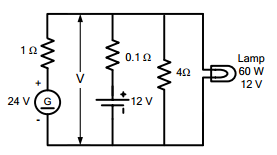A.  12.34 V

B.  11.24 V

C.  12.19 V

D.  11.66 V

Solution:

416.  EE Board Exam April 1982

Referring to the circuit diagram below, if the charger voltage  is  130  volts and the battery  voltage  is  120 volts, solve for the current Ib.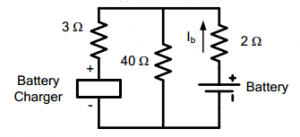A.  -0.215 A

B.  0.215 A

C.  -0.306 A

D.  0.306 A

Solution:

417.  EE Board Exam August 1977

In the figure below R1  = 1 ohm, R2  = 1 ohm, R3  = 3 ohms, I2 = 2 A and VB = 120 V. Find Eg.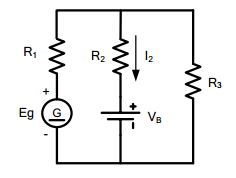A.  182.41 V

B.  153.32 V

C.  164.67 V

D.  157.22 V

Solution:

418.  EE Board Exam October 1980, April 1984

In  the  dc  circuit  as  shown,  the  high  resistance voltmeter gives a reading of 0.435 volt. What is the value of the resistance R?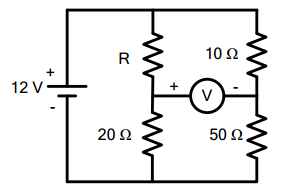A.  4 ohms

B.  5 ohms

C.  3 ohms

D.  2 ohms

Solution:

419.  EE Board Exam April 1980

Determine I in the figure.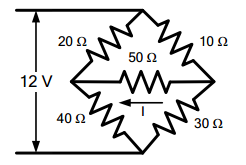A.  0.028 A

B.  0.010 A

C.  0.025 A

D.  0.014 A

Solution:

420.  ECE Board Exam April 1999

In  Kirchhoff’s  current  law,  which  terminal  of  a resistance  element  is  assumed  to  be  at  a  higher potential (more positive) than the other?

A.  The  terminal  where  the  current  exits  the resistance elements

B.  The  terminal  where  the  current  enters  the resistance elements

C.  Either A or B can be arbitrarily selected

D.  The  terminal  closest  to  the  node  being analyzed

Solution:

421.  ECE Board Exam April 2000

According  to  Kirchhoff’s  current  law,  what  is  the algebraic sum of all currents entering and exiting a node.

A.  zero

B.  a negative value

C.  the algebraic sum of all currents

D.  a positive value

Solution:

422.  ECE Board Exam November 1997

Find  the  Thevenin’s  impedance  equivalent  across R2 of a linear close circuit having 10 volts supply in series with  the resistors (R1  =  100 ohms and R2 =200 ohms).

A.  6.66 ohms

B.  6.666 kohms

C.  66.6 ohms

D.  666 ohms

Solution:

423.  ECE Board Exam April 1999

What is a node?

A.  A terminal point for a loop current

B.  A  connection  point  between  two  or  more conductors

C.  A formula

D.  A mathematical fiction

Solution:

424.  ECE Board Exam November 1998

If  a  resistance  element  is  part  of  two  loops,  how many  voltage  drops  must  be  calculated  for  that component?

A.  Two

B.  Three

C.  One

D.  None

Solution:

425.  ECE Board Exam April 1998

How many nodes are needed to completely analyze a circuit according to Kirchhoff’s current law?

A.  One

B.  Two

C.  One less than the total number of nodes in the circuit

D.  All nodes in the circuit

Solution:

426.  ECE Board Exam November 1996

Find  the  Thevenin’s  impedance  equivalent  across R2  of a linear close circuit having 10 volt supply in series with two resistors (R1=50 ohms and R2 = 200 ohms).

A.  400 ohms

B.  40 ohms

C.  4 ohms

D.  4 kohms

Solution:

427.  ECE Board Exam November 1995

In order to match the load to the generator means making load resistance ______.

A.  lower than generator’s internal resistance

B.  increased  to  more  generator’s  internal resistance

C.  decreased

D.  equal to generator’s internal resistance

Solution:

428.  A  circuit  contains  a  5  A  current  source  in  parallel with  an  8  ohm  resistor.  What  is  the  Thevenin’s voltage and Thevenin’s resistance of the circuit?

A.  40 V, 8 Ω

B.  5/8 V, 40 Ω

C.  5 V, 8 Ω

D.  5/8 V, 8 Ω

Solution:

429.  In the Norton’s equivalent circuit, the source is a

A.    constant voltage source

B.    constant current source

C.  constant voltage, constant current

D.  none of these

Solution:

430.  The  superposition  theorem  requires  as  many circuits to be solved as there are

A.    meshes

B.    source

C.  nodes

D.  all of these

Solution:

431.  Three resistors of 6-ohm resistance are connected in  delta.  Inside  the  delta  another  6-ohm  resistors are  connected  in  wye. Find  its  resistance between any two corners.

A.  2 ohms

B.  3 ohms

C.  4 ohms

D.  1 ohm

Solution:

432.  A  2-wire  dc  distribution  line  has  sending  end voltage  of  240  V  and  total  line  resistance  of  0.4 ohm. The maximum kW that can be transmitted by the line is ____.

A.  108

B.  72

C.  36

D.  144

Solution:

433.  An active element in a circuit is one which ____.

B.  supplies energy

C.  both receives and supplies energy

D.  none of these

Solution:

434.  An passive element in a circuit is one which ____.

A.  supplies energy

C.   both receives and supplies energy

D.  none of these

Solution:

435.  An electric circuit contains ____.

A.  active elements only

B.  passive element only

C.  both active and passive elements

D.  none of these

Solution:

436.  A  linear  circuit  is  one  whose  parameters  (e.g. resistances etc.) ____.

A.  change with change in current

B.  change with change in voltage

C.   do  not  change  with  change  in  voltage  and current

D.  none of these

Solution:

437.  In the circuit shown in Fig. 3.1, the number of nodes is ____.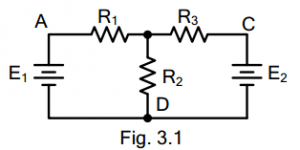A.  one

B.  two

C.  three

D.  four

Solution:

438.  In  the  circuit  shown  in  Fig.  3.1,  there  are  ____ junctions.A.  three

B.  four

C.  two

D.  none of these

Solution:

439.  The circuit shown in Fig. 3.1 has ____ branches.A.  two

B.  four

C.  three

D.  none of these

Solution:

440.  The circuit shown in Fig. 3.1 has ____ loops.A.  two

B.  four

C.  three

D.  none of these

Solution:

441.  In  the  circuit  shown  in  Fig.  3.1,  there  are  ___ meshes.A.  two

B.  three

C.  four

D.  none of these

Solution:

442.  To solve the circuit shown in Fig. 3.2 by Kirchhoff’s laws, we require ______.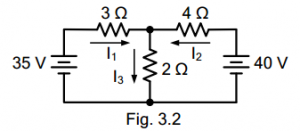A.  one equation

B.  two equations

C.  three equations

D.  four equations

Solution:

443.  To  solve  the  circuit  shown  in  Fig.  3.2  by  nodal analysis, we require ____A.  one equation

B.  two equation

C.  three equations

D.  none of these

Solution:

444.  To  solve  the  circuit  shown  in  Fig.  3.2  by superposition theorem, we require ____A.  one circuit

B.  two circuits

C.  three circuits

D.  none of these

Solution:

445.  To solve the circuit shown in Fig. 3.2 by Maxwell’s mesh current equation, we requireA.  one equation

B.  three equations

C.  two equations

D.  none of these

Solution:

446.  In the circuit shown in Fig. 3.3, the voltage at node B wrt D is calculated to be 15 V. The current in the 3 Ω resistor will be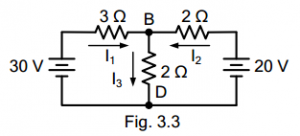A.  2 A

B.  5 A

C.  2.5 A

D.  none of these

Solution:

447.  The current in 2 Ω horizontal resistor in Fig. 3.3 is ____.A.  10 A

B.  5 A

C.  2 A

D.  2.5 A

Solution:

448.  In  order  to  solve  the  circuit  shown  in  Fig.  3.3  by nodal analysis, we requireA.  one equation

B.  two equation

C.  three equations

D.  none of these

Solution:

449.  The superposition theorem is used when the circuit contains

A.  a single voltage source

B.  a number of passive source

C.  passive elements only

D.  none of these

Solution:

450.  Fig.  3.4(b)  shows  the  Thevenin’s  equivalent  circuit of Fig. 3.4(a). The value of Thevenin’s voltage Vth is ____.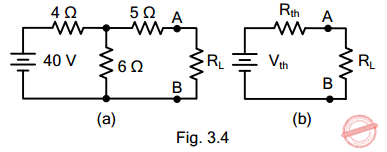A.  20 V

B.  24 V

C.  12 V

D.  36 V

Solution:

#### Questions and Answers in DC Circuits

Following is the list of practice exam test questions in this brand new series:

MCQ in DC Circuits
PART 1: MCQ from Number 1 – 50                Answer key: included
PART 2: MCQ from Number 51 – 100            Answer key: included
PART 3: MCQ from Number 101 – 150           Answer key: included
PART 4: MCQ from Number 151 – 200           Answer key: included
PART 5: MCQ from Number 201 – 250           Answer key: included
PART 6: MCQ from Number 251 – 300           Answer key: included
PART 7: MCQ from Number 301 – 350           Answer key: included
PART 8: MCQ from Number 351 – 400            Answer key: included
PART 9: MCQ from Number 401 – 450            Answer key: included
PART 10: MCQ from Number 451 – 500          Answer key: included

P inoyBIX educates thousands of reviewers and students a day in preparation for their board examinations. Also provides professionals with materials for their lectures and practice exams. Help me go forward with the same spirit.

“Will you subscribe today via YOUTUBE?”

Subscribe

PinoyBIX Engineering. © 2014-2020 All Rights Reserved | How to Donate? |